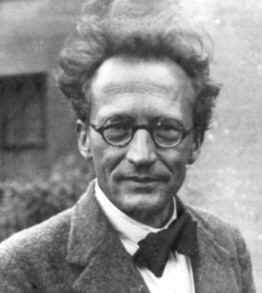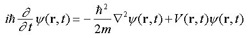A# Schrödinger, Erwin (1887–1961)Erwin Schrödinger played a principal part in the mathematical development of the modern model of the atom. He developed wave mechanics from de Broglie's picture of wave-particle duality.

Erwin Schrödinger was an Austrian theoretical physicist who first developed the version of quantum mechanics known as wave mechanics. In 1926, he put into mathematical form the revolutionary idea of the French physicist Louis Victor de Broglie that the motion of material particles is guided by so-called pilot waves. The formulation of the famous Schrödinger equation put quantum theory on a strict mathematical basis, and provided the foundation for its further rapid expansion. For this work, Schrödinger shared the 1933 Nobel Prize in Physics with Paul Dirac. Eventually it was shown that Schrödinger's wave mechanics were equivalent to the matrix mechanics of Werner Heisenberg.

In later years, Schrödinger concerned himself with the extension of Einstein's general theory of relativity to include electrical and magnetic phenomena. He also became interested in fundamental biology, and published a short, popular book, What Is Life? (1945). In this book, he attempted to explain the phenomena of life on the basis of purely physical concepts.

Schrödinger was born in Vienna, and in 1910 received his PhD from the university there. He served as a professor of theoretical physics in several universities in German and Switzerland. He was also associated for many years with the Dublin Institute for Advanced Studies.

## Schrödinger equation

Schrödinger equation's is an equation in quantum mechanics the solution of which gives the wavefunction (ψ) – a mathematical expression that contains all the information known about a particle. The time-dependent Schrödinger equation describes progressive waves and is applicable to the motion of free particles. The time-independent form describes standing waves.

Schrödinger's equation can be solved by the method of eigenvalues devised by Fourier. In this method, any mathematical function is expressed as the sum of an infinite series of other periodic functions. The trick is to find the correct functions that have the right amplitudes so that when added together by superposition they give the desired solution.

The time-dependent Schrödinger equation is:where t is time, r is displacement, m is the mass of the particle, i is the square root of minus one, and h-bar is Planck's constant divided by 2π.

## Schrödinger's cat

Schrödinger's cat is a paradoxical experiment in quantum mechanics devised by Schrödinger in 1935. It is a thought experiment designed as a response to the Copenhagen interpretation, which implies that subatomic particles can exist in two distinct states up until the moment they are observed. To demonstrate his own skepticism of the Copenhagen interpretation, Schrödinger conducted a hypothetical experiment using a Geiger counter, a radioactive substance, a flask, a hammer, some acid, a steel chamber, and a cat.

The cat is placed inside the chamber so it cannot be seen, along with the aforementioned items. If the radioactive substance decays sufficiently over the course of the test period, the hammer shatters the flask, which contains hydrocyanic acid (a poisonous gas), and the cat dies. But has the flask been shattered? Is the cat dead? While the chamber remains sealed, it is impossible to know. According to superposition and the Copenhagen interpretation designed to explain it, particles can simultaneously exist in all their possible states, therefore, the cat inside the chamber is, until observed, both alive and dead. But how long is a superposition supposed to exist? At what point does the poor cat become either demonstratively alive or very dead? The objective collapse theory says superpositions end spontaneously, meaning the cat will be alive or dead long before the chamber is opened. In any case just because something may occur on the subatomic level hardly means it can be replicated with a cat.